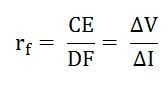# Resistance of a Diode

In practice, no diode is an Ideal diode, this means neither it acts as a perfect conductor when forward biased nor it acts as an insulator when it is reverse biased. In other words, an actual diode offers a very small resistance (not zero) when forward biased and is called a forward resistance.

Whereas, it offers a very high resistance (not infinite) when reverse biased and is called as a reverse resistance.

The various resistances of a diode are as follows:

##Forward Resistance

Under the forward biased condition, the opposition offered by a diode to the forward current is known as forward resistance. The forward current flowing through a diode may be constant, i.e., direct current or changing i.e., alternating current. The forward resistance is classified as Static Forward Resistance and Dynamic Forward Resistance.

### Static or DC Forward Resistance

The opposition offered by a diode to the direct current flowing forward bias condition is known as its DC forward resistance or Static Resistance. It is measured by taking the ratio of DC voltage across the diode to the DC current flowing through it.

The forward characteristic of a diode is shown below:It is clear from the graph that for the operating point P, the forward voltage is OA and the corresponding forward current is OB. Therefore, the static forward resistance of the diode is given as:### Dynamic or AC Forward Resistance

The opposition offered by a diode to the changing current flow I, in forward biased condition is known as its AC Forward Resistance. It is measured by a ratio of change in voltage across the diode to the resulting change in current through it.

From the figure A above, it is clear that for an operating point P the AC forward resistance is determined by varying the forward voltage (CE) on both the sides of the operating point equally and measuring the corresponding forward current (DF).

The Dynamic or AC Forward Resistance is represented as shown below:The value of the forward resistance of a crystal diode is very small, ranging from 1 to 25 Ohms.

## Reverse Resistance (RR)

Under the Reverse biased condition, the opposition offered by the diode to the reverse current is known as Reverse Resistance. Ideally, the reverse resistance of a diode is considered to be infinite. However, in actual practice, the reverse resistance is not infinite because diode conducts a small leakage current (due to minority carriers) when reverse biased.

The value of reverse resistance is very large as compared to forward resistance. The ratio of reverse to forward resistance is 1 00 000 : 1 for silicon diodes, whereas it is 40 000 : 1 for germanium diode.

### 7 thoughts on “Resistance of a Diode”

1. thanks for the info…was in need of it..and the language is pretty simple to understand and analyze..kudos

2. The dc resistance of a crystal diode is…….. it’s a.c. resistance.
1. the same as
2. more than
3. less than
4. none of the above